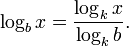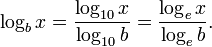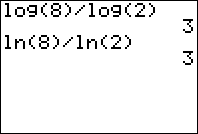# How to Enter Logarithms on Your Graphing Calculator

Filed under Difficulty: Easy, TI-83 Plus, TI-84 Plus, TI-89, TI-92 Plus.

Most graphing and scientific calculators have the ability to calculate logarithms, but you might come across questions which require you to use a different base than your calculator’s built-in functions. Thankfully, there is a simple formula called the “change of base” formula that allows you to calculate any logarithm on your calculator:Your calculator may have simply a or button, but for this formula you only need one of these:For example, to evaluate the logarithm base 2 of 8, enter ln(8)/ln(2) into your calculator and press ENTER. You should get 3 as your answer. Try it for yourself!### 7 Responses to How to Enter Logarithms on Your Graphing Calculator

1.zoeann anper

very helpful for a young smart mind like mine. In canada ( where i live) they don’t teach us how to use a dumb american invented calc. much love zoeann

•jay

American calc? its numbers, its not the calculator

•Anom

“american invented calc”
lul
the calculators are made by an american company yeah but all calculators are the same, they calculate numbers. log is a universal mathematical function, not just american, all calculators in the world (that are complex enough) have it

2.Milo

It would be much easier to input “log(8,2)”!

•jordan

dude, you are a genius.I hope people know that, you just helped me so much.

3.SilverWOLF

for the “k” part of the formula, can that be any number? Looks like yes to me because if numerator and denominator are log to base k, then it will be simplified, right?

•Deepthi

Ok nice